### Transient Vibrations: Response of Spring–Mass System to a Step Function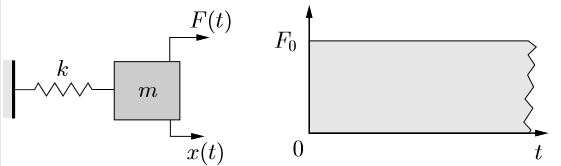(a) Schematic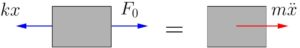(b) FBD/MAD (x, x¨ > 0)

Consider a simple spring–mass system subjected to a rectangular step load as shown in Figure 7.1(a). We would like to find the response of the mass to this transient loading condition. Figure 7.1(b) shows the associated FBD/MAD. The equation of motion obtained using Newton’s laws is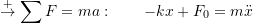or

(7.2)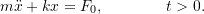As usual, the solution is composed of the homogeneous and particular solutions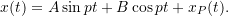For the RHS in (7.2), the particular solution is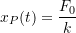so the total solution becomes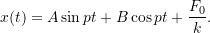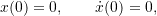the constants *Note that the total solution (including the particular solution) must be used to determine the arbitrary constants which arise from the homogeneous solution.* become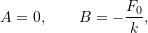so that the total solution is

(7.3)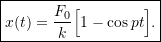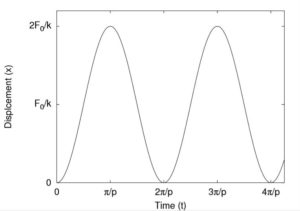Figure 7.2: Response of simple spring–mass system to applied step load

This response is illustrated in Figure 7.2. We can see that the maximum displacement of the mass is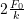which is twice the deflection that would have resulted if the load were applied statically. Now consider what happens if the step load is “turned off” at some time. After this time, since there is no longer any applied force, the system undergoes free vibrations given by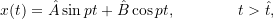where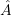and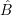need to be evaluated at the “initial” conditions (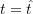) which depend on the timeat which the force was removed. The response of the mass while the step load is applied is given in equation (7.3). Similarly, the velocity of the mass during this period is, by differentiation,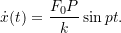Therefore, at the timewhen the load is removed, the “initial” conditions are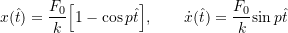which can be used to findand.

Consider two specific examples:

1.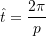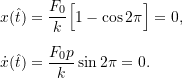As a result,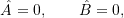so the response in this case is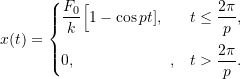1.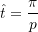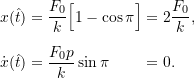As a result,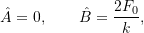so the response in this case is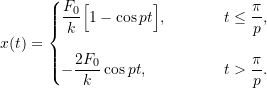These responses are shown in Figure 7.3. As these responses clearly show, the transient nature of the applied loading can have a significant effect on the resulting response.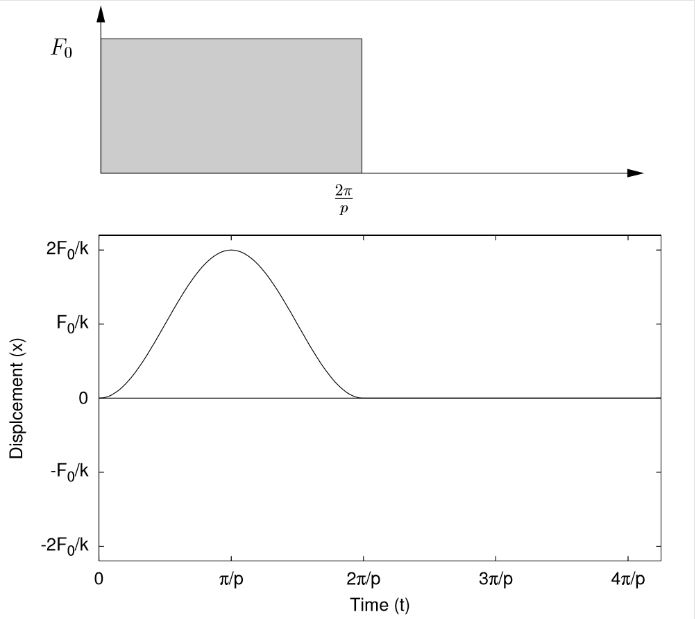(a) τ =2π/p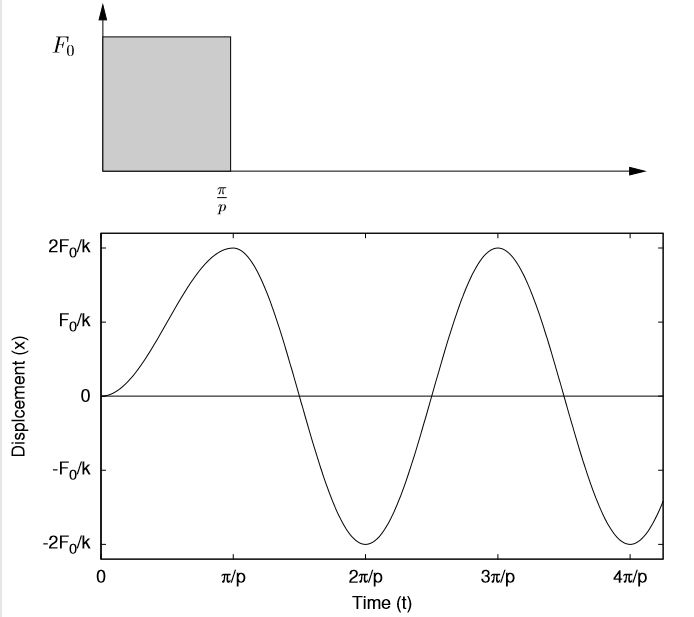(b) τ = π/p

Figure 7.3: Response of simple spring–mass system to applied step load which is subsequently removed

The previous responses can be understood on a physical basis. In the first case,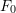is applied to a system originally in its equilibrium position. Whenis applied, the effect is to shift the new equilibrium position a distance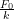as shown in Figure 7.4.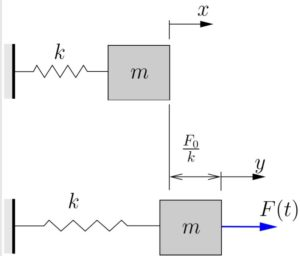Figure 7.4: Shift of equilibrium position

At time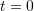(immediately after the application of), the mass is apparently in a position (top of Figure 7.4}) where it is displaced from its new equilibrium position. If we measure the displacement of the mass from the new equilibrium position as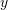and neglect both the applied forceand the new equilibrium stretch in the spring (as we did regularly when gravity was present), we then have a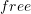vibration problem with the initial conditions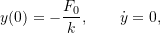so the free vibration response is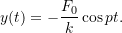However, since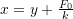, we can write the response in terms ofas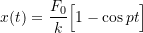as before.

The two cases shown in Figure 7.3 can be understood on similar principles. When the forceis removed, the equilibrium position of the system returns to its original position. The time at which the forceis removed determines the initial conditions for the subsequent free vibration problem, which leads to the responses shown.

### Response of Spring–Mass System to an Impulse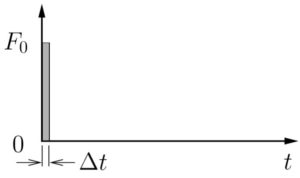We now consider the response of a spring–mass system subjected to an impulsive loading shown in Figure 7.5. (Note that the quantity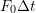is the area under the force-time curve and is known as the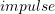applied to the system.) Here the timeat which the load is removed is small (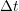) so that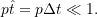In such a case,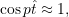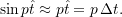The “initial” conditions are approximately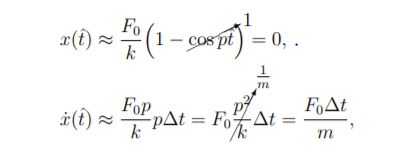so the initial conditions (after impulse has been applied) become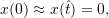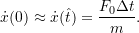The constantsandare then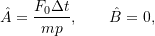so the response becomes

(7.4)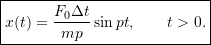This response will play an important role later when we consider the method of convolution.

#### Aside

We can obtain this result another way by applying the basic principle of impulse and momentum for our system.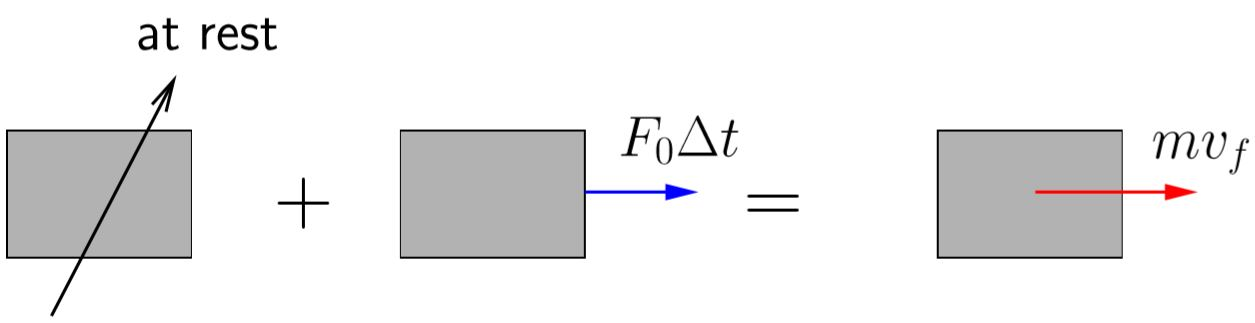Figure 7.6: Impulse–momentum diagram for mass m

Figure 7.6 shows an impulse–momentum diagram for our simple spring mass system when the impulsive load is applied. The system is initially at rest and after the impulse has been applied has a resulting velocity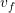. (Note that the force of the spring is not included in the diagram. The assumption is that the impulse is applied fast enough that the mass does not have time to move while the impulse is being applied. Since the mass does not move, the force in the spring remains unchanged. We say that the force of the spring is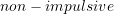.)
Applying the principle of impulse and momentum shows that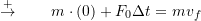so we get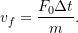After the impulse is over, we have a free vibration problem with the initial conditions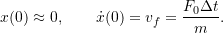The two constants are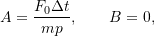so the response becomes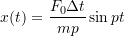as in equation (7.4).

We can also consider the response to some additional useful forcing functions.